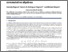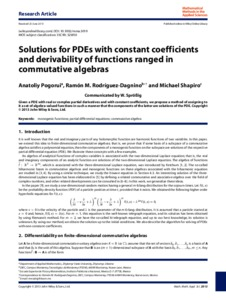Solutions for PDEs with constant coefficients and derivability of functions ranged in commutative algebras

Pogoruі, А. А. and Rodríguez-Dagnіno, Ramón М. and Shapіro, Мichael (2013) Solutions for PDEs with constant coefficients and derivability of functions ranged in commutative algebras. Math. Meth. Appl. Sci..Preview
Text

Abstract

It is well known that the real and imaginary parts of any holomorphic function are harmonic functions of two variables. In this paper, we extend this idea to finite-dimensional commutative algebras; that is, we prove that if some basis of a subspace of a commutative algebra satisfies a polynomial equation, then the components of a monogenic function on the subspace are solutions of the respective partial differential equation (PDE). We illustrate these concepts with a few examples.

Item Type: Article Q Science > QA Mathematics > Mathematical Analysis Faculty of Physics and Mathematics > Department of Mathematical Analysis Ірина Ігорівна Таргонська 23 Oct 2014 09:32 15 Aug 2015 09:15 http://eprints.zu.edu.ua/id/eprint/13298View Item